#### Schrödinger' Equation

Erwin Schrödinger was the first person to successfully apply the concept of the wave nature of matter to an explanation of electronic structure.
He developed another equation called the Schrödinger wave equation which allowed him to determine the energy levels and wave properties of the hydrogen atom, other atoms and molecules.
For a one partice system the equation takes the form: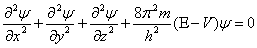where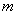is the mass of the particle,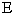and V are its total and potential energies, respectively,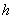is Plank’s constant, and the first three terms are partial derivatives with respect to x, y, and z, of the wave function,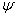, (Psi) to be associated with the particle.

The wave properties of matter form the foundation for the theory called wave mechanics, which serves as the basis of all current theories of electronic structure.

• The term quantum mechanics is also used because wave mechanics predicts quantized energy levels.
• The theory of quantum mechanics tells us that in the atom, electron waves are standing waves.
• The electrons can have different waveforms or wave patterns.
• Each of these waveforms which are called orbitals, is described in quantum mechanics by a mathematical expression called a wave function.
• The wave function can be used to describe the shape of the electron wave and its energy.
• Energy changes within an atom are simply the result of an electron changing from a wave pattern with one energy to a wave pattern with a different energy.

When an atom is in its most stable state, the ground state, the electrons of the atom have waveforms with the lowest possible energies.

The shapes of the wave patterns are important because the theory tells us that the amplitude of a wave at any particular place is related to the likelihood of finding the electron there.

Wave mechanics tells us that the three-dimensional waves, orbitals, can be characterized by three integer quantum numbers n, l, and ml.